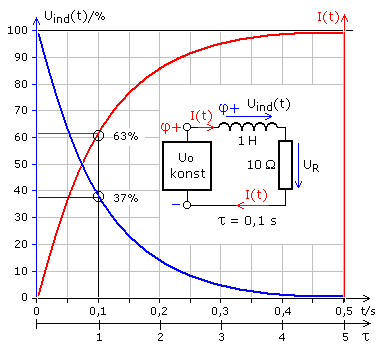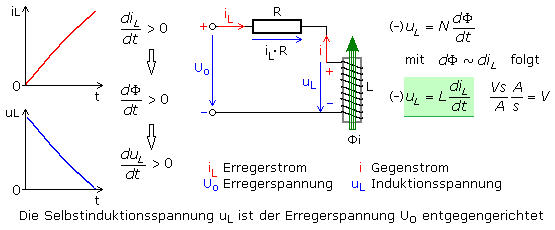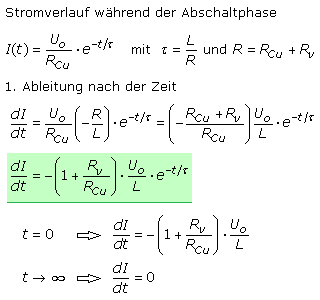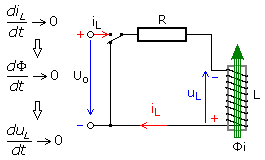# How can the output voltage be zero

## The coil in the DC circuit

A coil is the sequence of many loops of wire connected in series. They are usually wound on a mechanical carrier, the coil body often in several layers on top of each other. A coil always has an ohmic resistance. It is calculated from the electrical conductivity, the conductor wire, the wire length and the conductor cross-section. Compared to the properties of the inductance, the ohmic component has practically less influence. Further general properties of a coil are described in a separate chapter.

If a constant DC voltage is applied to a coil, voltage and current changes can be shown graphically with a two-channel oscilloscope. For the current measurement, a small ohmic resistor must be connected in series in order to display the current changes as proportional voltage changes. With many oscilloscopes, the reference ground of the channels is identical, so the value for the current measurement resistor should be small compared to the wire resistance of the coil. The evaluation initially shows a non-linear course of both measured variables, which assume constant values ​​after a certain time. The behavior of a coil in a DC circuit is thus comparable to the charging of a capacitor on a DC voltage source, with the difference that the coil stores the supplied energy in the magnetic field and the capacitor in the electric field.

### Circuit diagrams in the DC circuit

The following video clip shows the switch-on and switch-off processes for three different resistance and inductance values. The wire resistance of the coil is contained in the series resistor. The energy source supplies a constant DC voltage. The video clip can only be controlled with the help of the fade-in control bar.

If the circuit is closed, the current increases only slowly from zero. That seems to be unusual for a circuit with an ohmic wire resistor. Every real coil is a series connection of the ohmic wire resistance R with the ideal inductance L (coil). The circuit forms a mesh with the supply voltage in which the voltage sum is zero according to Kirchhoff's second rule. The voltage across the ohmic resistor always has the same polarity as the current flowing through it. The total voltage can only be zero if the voltage on the coil is polarized opposite to the supply voltage.The measurement diagram shows that immediately after switching on the voltage is maximum and the current is minimum. The coil must therefore generate a voltage that is almost as high as the applied DC supply voltage. The upper coil connection must have positive potential. Only then can it counteract the current that wants to flow through the circuit from the applied voltage. The low inrush current increases over time and the voltage across the coil decreases. The process can be described mathematically with an exponential function (exponential function). After a certain time, a constant current flows which is calculated from the DC supply voltage and the wire resistance of the coil.

In DC voltage operation, almost no voltage is measured parallel to the coil, if it has a very low wire resistance. The time until this stable final state is reached depends on the value of the wire resistance of the coil and, if available, an additional series resistor. The greater the ohmic value in this series connection, the faster the final state is reached. In practice it is reached after five time constants (5 τ). The time constant tau is defined as τ = L / R the ratio of the inductance L and the ohmic series resistance R.

The time constant τ is directly proportional to the inductance and inversely proportional to the switching resistance of the coil.
After 1 τ the inrush current has reached 63% of its final value. The switching time of a coil is 5 τ.

### The series connection of coils

If several inductances are connected in series in a circuit and their magnetic fields do not influence each other, the total inductance is equal to the sum of the individual values.

L = L1 + L2 + ... + Ln

### The parallel connection of coils

Assuming that their magnetic fields do not influence each other, the total inductance for inductors connected in parallel is calculated as follows:### The magnetic energy of a coil

A real coil connected to DC voltage receives electrical energy. Part of the resistance is converted into heat. The greater part is stored in the magnetic field. Since it is a non-linear dynamic process, only differentially small time segments can be expected. The ultimate relationship comes through integration.Further information on the coil in the AC circuit can be found on the page: AC resistances, inductive reactance.

### The switch-on behavior and the self-induced voltage

If electrical current flows through a coil, a magnetic flux is created around every turn of the conductor and the coil builds up a magnetic field. The magnetic flux changes proportionally to the change in current. Just as an induction voltage is generated by external magnetic excitation at the coil ends, it also reacts to the changing magnetic field that is created by an impressed current. The coil generates the self-induction voltage and becomes the voltage source. According to Lenz's rule, the self-induction voltage UL is directed in such a way that it counteracts the change in current.At the moment of switch-on, the change in current is greatest and the self-induction voltage is almost the same as the generator voltage. If Lenz's rule were not met, the circuit would generate additional energy on its own. It is still true that energy cannot be generated or destroyed, but can only be converted into other forms of energy.

Until the maximum current is reached, the coil builds up its magnetic field and stores magnetic energy. During this time the current IL increases from the value zero according to a mathematical exponential function to the final value (U / R). The rate of change of the current dI / dt practically reaches the value zero after 5 τ. This is followed by the static state with constant current, which is determined by the operating voltage and the ohmic circuit resistance. In a comparable way, the self-induced voltage decreases according to an exponential function from the initial maximum value towards zero.

### Mathematical derivations

The measurement protocols show that at time t = 0 the current I (t) = 0. It should be a real coil, i.e. a series connection of ideal inductance L and wire resistance RCu. The current course over time is described by an exponential function. The exponent contains the ratio t / τ as a pure numerical value. The exponent must be negative so that a constant current is achieved after some time. The change in current over time is determined using the 1st derivative of the exponential function.The limit value observation shows that the current change is greatest at the moment the device is switched on and tends towards zero after the magnetic field has built up. If the magnetic field of a coil is built up with a current change that is constant over time, then the induction voltage changes proportionally. According to Lenz's rule, the polarity is opposite to the voltage causing it. The constant of proportionality is the inductance L of the coil. The voltage curve for the switch-on process can thus be derived mathematically.The limit value observation confirms the measurement results. The absolute value of the induction voltage is equal to the applied DC operating voltage at time t = 0. As a self-induction voltage, the sign is opposite to that of the operating voltage. If the magnetic field no longer changes after five time constants with dI / dt = 0, then the self-induced voltage Uind = 0.

The course of the (self) induction voltage is determined by the wire resistance or by the total resistance of the series resistor and wire resistance. After switching on, the voltage changes at the resistor result from the sum of the operating voltage and the self-induced voltage. This can be written mathematically as follows.If the magnetic field is practically completely built up after 5 · τ, the maximum current determined by the operating voltage and the resistance value flows. Without a series resistor, the coil would almost short-circuit the DC voltage source.

### The shutdown behavior

The video clip or the measurement protocols for laboratory or simulation tests show that the switch-on process is completed after five time constants. The coil has completely built up its magnetic field, stores energy in it and does not generate any self-induced voltage. According to Ohm's law, the maximum constant current flowing in the circuit is only dependent on the supply voltage and the wire resistance of the coil and any existing series resistor. No voltage would be measurable on an ideal coil. If the voltage source is switched off and the circuit is closed to ground, the measurements show that the current continues to flow in the same direction as before. Starting from the maximum value, it decreases exponentially from this point in time t = 0 to the value zero.When the supply voltage is switched off, the coil counteracts the loss of the supply current according to the induction law and Lenz's rule. The magnetic field receives no energy and the field strength decreases. To counteract this decrease, the missing excitation current must be compensated. With the help of the magnetic field, the coil generates a voltage which, according to Lenz's rule, is polarized so that the current can continue to flow in the original direction. The polarity of the (self) induced voltage is the same as that of the supply voltage. It is opposite to the polarity of the self-induction voltage during the switch-on process. If the magnetic field is reduced after five time constants, the current and voltage have reached their final value of zero.

### Mathematical derivations

At the moment of switch-off, the current that is calculated from the supply voltage and the wire resistance RCu of the coil flows. To close the circuit, an external resistor Rv is connected in series with the wire resistor. The total resistance R of the circuit now acts in the time constant. The current decreases according to an exponential function and the change in current over time is determined using the 1st derivative of this function.The minus sign indicates the current decrease and the decrease speed is determined by the series resistor. It is in the counter and the larger its value, the faster the current will decrease. The change in the induction voltage can be derived using the same approach that was used above for the switch-on torque.The limit value observations confirm the experimental observations. The induction voltage is highest at the moment of switch-off and depends on the discharge resistance. The larger this value, the higher the voltage peak. When switching off, the following general equations apply to the current and voltage curves.### Protection against high induction voltages when switching off

The cut-off voltage can be measured with a simulation program. A coil with 100 mH and 10 Ω wire resistance was connected to U = 1 V direct voltage. After reaching the maximum direct current I = 100 mA, the circuit was interrupted and the coil was switched to ground with a discharge resistor R = 90 Ω. At the time of the interruption, the induction voltage on the coil is calculated to be 10 V. The simulation confirms the result. The time constant for the switch-off phase is τ = 1 ms.With the same 1 V operating voltage but a discharge resistance of 990 Ω, the voltage peak reaches 100 V at the moment of switch-off. Load circuits are often switched with relays, whose transistorized control circuit is carried out with a low voltage of 12 to 24 V and an operating current of 50 mA. If the relay is switched off, the transistor has an extremely high resistance. The voltage peak of the coil can reach values ​​that are above the permitted collector-emitter reverse voltage. To protect the transistor, a so-called freewheeling diode is connected in parallel to the relay coil. It is switched in the reverse direction for the DC operating voltage of the transistor circuit. At the moment of switch-off, the diode becomes conductive and limits the voltage peak at the coil to <1 V.

If larger inductances are switched with mechanical switches, the shutdown resistance is determined by the insulation resistance between the contacts. If at least 1 MΩ is assumed for air, then the switch-off peak is many kilovolts. Dry air insulates around 3 kV per millimeter. Without protective measures, spark gaps with contact erosion form or they weld together. The contacts can be protected in AC operation with a resistor connected in parallel or an RC series combination. In DC operation, a free-wheeling diode connected in anti-parallel is well suited.

### Gustav Robert Kirchhoff ✖

The German physicist Kirchhoff (1824-1887) worked as a professor in Breslau from 1850-54, in Heidelberg from 1854-75 and in Berlin from 1875-86. As early as 1845 as a student in Königsberg, he formulated the laws of current branching in a general form, which are known today as Kirchhoff's 1st and 2nd rule. He examined the electricity line and realized that it was running almost at the speed of light (1857).

Together with R. W. Bunsen he researched the basics of spectral analysis. Both researchers explained the Frauenhofer absorption lines in the solar spectrum. They found the elements cesium and rubidium. Kirchhoff also dealt with questions of mechanics and acoustics and provided the explanations for the Chladni figures.

### Knot rule - 1. Kirchhoff's rule

In each branching point, the node, the sum of the inflowing currents is equal to the sum of the outflowing currents. In general, the following applies to I:### Mesh rule - 2. Kirchhoff's rule

In every closed circuit, the mesh, the sum of the electromotive forces, the original voltages U, is equal to the sum of all voltage drops, equal to the products I · R (I current, R resistance). In general, the following applies to U:### Heinrich Friedrich Emil Lenz ✖

Lenz's rule is named after the Russian physicist of German origin H. Lenz (1804 - 1865). It defines the direction of the current that is induced by a time-varying magnetic field. According to this rule, the induction current is directed in such a way that its own magnetic field counteracts the cause of the induction.

H. Lenz also dealt with the Peltier effect and was able to freeze water with this method.